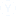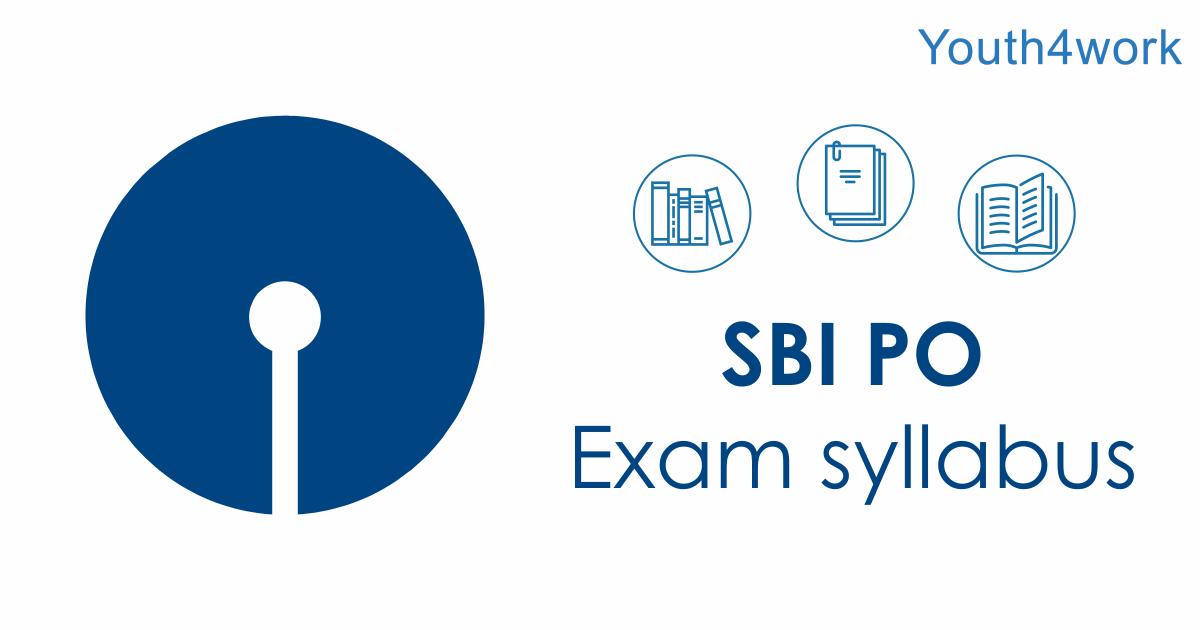# SBI Prem Practice REAONING Q2

Read the following information carefully and answer the questions, which follow : ‘A – B’ means ‘A is father of B’. ‘A + B’ means ‘A is daughter of B’. ‘A ÷ B’ means ‘A is son of B’. ‘A × B’ means ‘A is wife of B’. FOR QUESTION PLEASE CLICK THE FORUM

QUESTIONS

6. How is P related to T in the expression ‘P + S – T’?
1) Sister
2) Wife
3) Son
4) Daughter
5) None of these

7. In the expression ‘P × Q – T’ how is T related to P?
1) Daughter
2) Sister
3) Mother
4) Can’t be determined
5) None of these

8. Which of the following means T is wife of P?
1) P × S ÷ T
2) P ÷ S× T
3) P – S ÷ T
4) P + T ÷ S
5) None of these
READ MORE : SBI PRACTICE 3
9. Which of the following means P is grandson of S?
1) P + Q – S
2) P ÷ Q × S
3) P ÷ Q + S
4) P × Q ÷ S
5) None of these
READ MORE : SBI PRACTICE 4
10. In the expression ‘P + Q × T’ how is T related to P?
1) Mother
2) Father
3) Son
4) Brother
5) None of these

7• sistet

• 3

• none of this

• can't determine

• sister

• father

• option 3

• none of these

• daughter

• sister

• none of this

• sisters

• Sister

• None of these

• mother

• sister

• sister

• 2

• 2

• 3Other Related DiscussionsPractice Mock Test
sbi po prelim exam